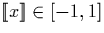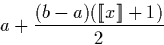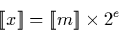Next: Nested interval Representation Up: Representations Previous: Dyadic Rational Streams

# (Mantissa, Exponent) Representation

The signed binary and dyadic digit streams described in sections 3.3.1 and 3.3.2 represent reals in the range [-1,1]. In fact the choice of [-1,1] as endpoints is arbitrary, as we could easily interpret a given stream as a real in any closed interval.

To illustrate, suppose we have a numeral x which we would interpreted as the number. We could just as easily interpret x as a number in the range [a,b] asWhen it is necessary to represent reals on the whole real line, however, the stream representation alone is not sufficient. Perhaps the simplest solution to this problem is to introduce a (mantissa, exponent) representation similar to a floating point number. We do this using a mantissa which is a stream of either signed binary or dyadic digits, and and exponent is an integer of unrestricted size. For simplicity, the exponent represents a power of two, although powers of other numbers could also be used.

Hence, given a numeral x with mantissa m and exponent e, we have:Most algorithms for operations on reals represented using a mantissa and exponent in this way reduce to two simple and independent operations on the mantissa and exponent.Next: Nested interval Representation Up: Representations Previous: Dyadic Rational Streams
Martin Escardo
5/11/2000